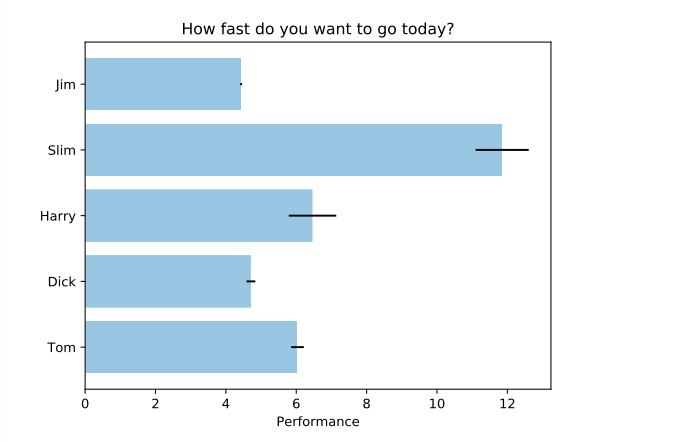# Using matplotlib¶

Matplotlib is a multi-platform data visualization library, which you can use to graphically represent your datasets.

Perform the following steps to generate matplotlib visuals:

1. Navigate to the Notebooks page.

2. Enter the matplotlib code in the paragraph and click the Run button.

Note

If the language of the notebook is not pyspark, then you must use `%pyspark` as the first line in each paragraph.

The following example shows a sample code.

```import matplotlib
import numpy as np
import matplotlib.pyplot as plt
# Example data
people = ('Tom', 'Dick', 'Harry', 'Slim', 'Jim')
np.random.seed(1234)
y_pos = np.arange(len(people))
performance = 3 + 10 * np.random.rand(len(people))
error = np.random.rand(len(people))

plt.barh(y_pos, performance, xerr=error, align='center', alpha=0.4)
plt.yticks(y_pos, people)
plt.xlabel('Performance')
plt.title('How fast do you want to go today?')

z.showplot(plt)
```

The `z.showplot()` function in the sample code is a Qubole specific function that is used to plot the graphs.

The respective graph is displayed in the Notebooks page as shown in the following figure.